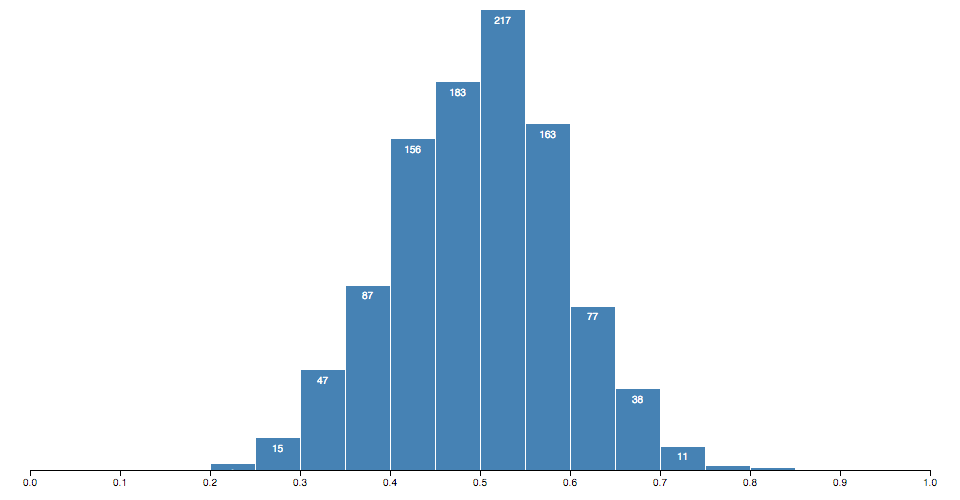# # d3-array

JavaScript 中的数据通常由一个数组来表示, 所以当可视化或分析数据时往往也会操作数组. 常见的数组操作包括切片, 过滤, 遍历等等. JavaScript 本身支持的数组操作可以参考 这里 (opens new window).

JavaScript 中 修改数组自身 的方法:

JavaScript 中数组的 存取方法 :

JavaScript 中数组的 迭代方法 :

## # Installing

NPM 安装: `npm install d3-array`. 或者下载 latest release (opens new window). 也可以直接从 d3js.org (opens new window)standalone library (opens new window) 或作为 D3 4.0 (opens new window) 的一部分载入. 支持 AMD, CommonJS 以及最基本的标签引入形式, 如果使用标签引入会暴露全局 `d3` 变量:

``````<script src="https://d3js.org/d3-array.v1.min.js"></script>
<script>

var min = d3.min(array);

</script>
``````

## # API Reference

### # Statistics

# d3.min(array[, accessor]) <源码> (opens new window)

# d3.max(array[, accessor]) <源码> (opens new window)

# d3.extent(array[, accessor]) <源码> (opens new window)

# d3.sum(array[, accessor]) <源码> (opens new window)

# d3.mean(array[, accessor]) <源码> (opens new window)

# d3.median(array[, accessor]) <源码> (opens new window)

# d3.quantile(array, p[, accessor]) <源码> (opens new window)

``````var a = [0, 10, 30];
d3.quantile(a, 0); // 0
d3.quantile(a, 0.5); // 10
d3.quantile(a, 1); // 30
d3.quantile(a, 0.25); // 5
d3.quantile(a, 0.75); // 20
d3.quantile(a, 0.1); // 2
``````

# d3.variance(array[, accessor]) <源码> (opens new window)

# d3.deviation(array[, accessor]) <源码> (opens new window)

# d3.scan(array[, comparator]) <源码> (opens new window)

``````var array = [{foo: 42}, {foo: 91}];
d3.scan(array, function(a, b) { return a.foo - b.foo; }); // 0
d3.scan(array, function(a, b) { return b.foo - a.foo; }); // 1
``````

# d3.bisectLeft(array, x[, lo[, hi]]) <源码> (opens new window)

# d3.bisect(array, x[, lo[, hi]]) <源码> (opens new window)
# d3.bisectRight(array, x[, lo[, hi]]) <源码> (opens new window)

bisectLeft 操作类似, 但是遍历方向是自右向左, 如果 xarray 中的值一样则插入点会定位在已有元素的右侧. 返回的插入点会将原数组分为两部分: v <= x 的值会包含在 array.slice(lo, i) 中(左侧半部分), v > x 包含在 array.slice(i, hi) 中(右半部分).

# d3.bisector(accessor) <源码> (opens new window)
# d3.bisector(comparator) <源码> (opens new window)

``````var data = [
{date: new Date(2011, 1, 1), value: 0.5},
{date: new Date(2011, 2, 1), value: 0.6},
{date: new Date(2011, 3, 1), value: 0.7},
{date: new Date(2011, 4, 1), value: 0.8}
];
``````

``````var bisectDate = d3.bisector(function(d) { return d.date; }).right;
``````

``````var bisectDate = d3.bisector(function(d, x) { return d.date - x; }).right;
``````

# bisector.left(array, x[, lo[, hi]]) <源码> (opens new window)

# bisector.right(array, x[, lo[, hi]]) <源码> (opens new window)

# d3.ascending(a, b) <源码> (opens new window)

``````function ascending(a, b) {
return a < b ? -1 : a > b ? 1 : a >= b ? 0 : NaN;
}
``````

# d3.descending(a, b) <源码> (opens new window)

``````function descending(a, b) {
return b < a ? -1 : b > a ? 1 : b >= a ? 0 : NaN;
}
``````

### # Transformations

# d3.cross(a, b[, reducer]) <源码> (opens new window)

``````function pair(a, b) {
return [a, b];
}
``````

``````d3.cross([1, 2], ["x", "y"]); // returns [[1, "x"], [1, "y"], [2, "x"], [2, "y"]]
d3.cross([1, 2], ["x", "y"], (a, b) => a + b); // returns ["1x", "1y", "2x", "2y"]
``````

# d3.merge(arrays) <源码> (opens new window)

``````d3.merge([, [2, 3]]); // returns [1, 2, 3]
``````

# d3.pairs(array[, reducer]) <源码> (opens new window)

``````function pair(a, b) {
return [a, b];
}
``````

``````d3.pairs([1, 2, 3, 4]); // returns [[1, 2], [2, 3], [3, 4]]
d3.pairs([1, 2, 3, 4], (a, b) => b - a); // returns [1, 1, 1];
``````

# d3.permute(array, indexes) <源码> (opens new window)

``````var object = {yield: 27, variety: "Manchuria", year: 1931, site: "University Farm"},
fields = ["site", "variety", "yield"];

d3.permute(object, fields); // returns ["University Farm", "Manchuria", 27]
``````

# d3.shuffle(array[, lo[, hi]]) <源码> (opens new window)

# d3.ticks(start, stop, count) <源码> (opens new window)

startstop (包含)之间返回大约 count + 1 个等间隔的数组序列, 每个值都是 10 的 1, 2 或 5 的次幂. 参考 d3.tickIncrement, d3.tickSteplinear.ticks (opens new window).

# d3.tickIncrement(start, stop, count) <源码> (opens new window)

d3.tickStep 类似, 但是要求 start 总是小于等于 step, 如果给定的 start, stop 以及 count 计算出来的步长小于 1 时则对步长求反. 这个方法始终保持返回整数, 并且被 d3.ticks 使用以避免生成不精确的浮点数. 与 d3.tickStep 类似，除了要求 start 始终小于或等于 step，如果根据给定的 start, stopcount 计算出来的 `tick` 步长小于 `1` 则返回负值. 这个方法永远保证返回一个整数提供给 d3.ticks, 避免浮点数达到精确表示的目的.

# d3.tickStep(start, stop, count) <源码> (opens new window)

# d3.range([start, ]stop[, step]) <源码> (opens new window)

``````d3.range(0, 1, 0.2) // [0, 0.2, 0.4, 0.6000000000000001, 0.8]
``````

``````d3.range(0, 1, 1 / 49); // BAD: returns 50 elements!
d3.range(49).map(function(d) { return d / 49; }); // GOOD: returns 49 elements.
``````

# d3.transpose(matrix) <源码> (opens new window)

# d3.zip(arrays…) <源码> (opens new window)

``````d3.zip([1, 2], [3, 4]); // returns [[1, 3], [2, 4]]
``````

### # Histograms(opens new window)

# d3.histogram() <源码> (opens new window)

# histogram(data) <源码> (opens new window)

• `x0` - 当前分箱的最小值(包含).
• `x1` - 当前分箱的最大值(不包含, 除非是最后一个分箱).

# histogram.value([value]) <源码> (opens new window)

# histogram.domain([domain]) <源码> (opens new window)

``````var histogram = d3.histogram()
.domain(x.domain())
.thresholds(x.ticks(20));
``````

``````var bins = histogram(numbers);
``````

# histogram.thresholds([count]) <源码> (opens new window)
# histogram.thresholds([thresholds]) <源码> (opens new window)

### # Histogram Thresholds

# d3.thresholdFreedmanDiaconis(values, min, max) <源码> (opens new window)

# d3.thresholdScott(values, min, max) <源码> (opens new window)

# d3.thresholdSturges(values) <源码> (opens new window)